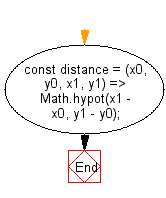# JavaScript: Get the distance between two given points

## JavaScript fundamental (ES6 Syntax): Exercise-243 with Solution

Write a JavaScript program to get the distance between two given points.

• Use Math.hypot() to calculate the Euclidean distance between two points.

Sample Solution:

JavaScript Code:

``````//#Source https://bit.ly/2neWfJ2
const distance = (x0, y0, x1, y1) => Math.hypot(x1 - x0, y1 - y0);
console.log(distance(1, 1, 2, 3));
console.log(distance(-1, -1, 2, 3));
```
```

Sample Output:

```2.23606797749979
5
```

Flowchart:Live Demo:

See the Pen javascript-basic-exercise-243-1 by w3resource (@w3resource) on CodePen.

Improve this sample solution and post your code through Disqus

What is the difficulty level of this exercise?

Test your Programming skills with w3resource's quiz.

﻿